# Dihedral group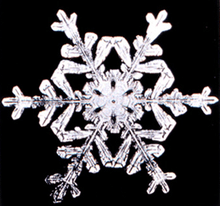The symmetry group of a snowflake is D6, a dihedral symmetry, the same as for a regular hexagon.

In mathematics, a dihedral group is the group of symmetries of a regular polygon, which includes rotations and reflections. Dihedral groups are among the simplest examples of finite groups, and they play an important role in group theory, geometry, and chemistry.

The notation for the dihedral group of order n differs in geometry and abstract algebra. In geometry, Dn or Dihn refers to the symmetries of the n-gon, a group of order 2n. In abstract algebra, Dn refers to the dihedral group of order n. The geometric convention is used in this article.

## Definition

### Elements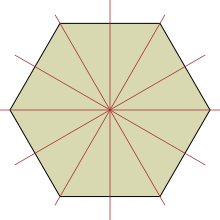The six axes of reflection of a regular hexagon

A regular polygon withsides hasdifferent symmetries:rotational symmetries andreflection symmetries. The associated rotations and reflections make up the dihedral group. Ifis odd, each axis of symmetry connects the midpoint of one side to the opposite vertex. Ifis even, there are n/2 axes of symmetry connecting the midpoints of opposite sides andaxes of symmetry connecting opposite vertices. In either case, there areaxes of symmetry andelements in the symmetry group. Reflecting in one axis of symmetry followed by reflecting in another axis of symmetry produces a rotation through twice the angle between the axes.

The following picture shows the effect of the sixteen elements ofon a stop sign: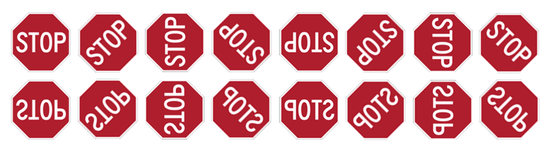The first row shows the effect of the eight rotations, and the second row shows the effect of the eight reflections, in each case acting on the stop sign with the orientation as shown at the top left.

### Group structure

As with any geometric object, the composition of two symmetries of a regular polygon is again a symmetry of this object. With composition of symmetries to produce another as the binary operation, this gives the symmetries of a polygon the algebraic structure of a finite group.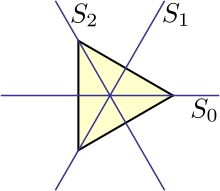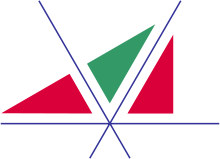The composition of these two reflections is a rotation.

The following Cayley table shows the effect of composition in the group D3 (the symmetries of an equilateral triangle). r0 denotes the identity; r1 and r2 denote counterclockwise rotations by 120° and 240° respectively, and s0, s1 and s2 denote reflections across the three lines shown in the adjacent picture.

r0r1r2s0s1s2
r0 r0 r1 r2 s0 s1 s2
r1 r1 r2 r0 s1 s2 s0
r2 r2 r0 r1 s2 s0 s1
s0 s0 s2 s1 r0 r2 r1
s1 s1 s0 s2 r1 r0 r2
s2 s2 s1 s0 r2 r1 r0

For example, s2s1 = r1, because the reflection s1 followed by the reflection s2 results in a rotation of 120°. The order of elements denoting the composition is right to left, reflecting the convention that the element acts on the expression to its right. The composition operation is not commutative.

In general, the group Dn has elements r0, …, rn−1 and s0, …, sn−1, with composition given by the following formulae:In all cases, addition and subtraction of subscripts are to be performed using modular arithmetic with modulus n.

### Matrix representation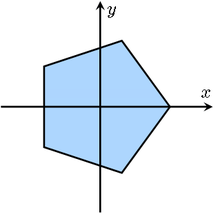The symmetries of this pentagon are linear transformations of the plane as a vector space.

If we center the regular polygon at the origin, then elements of the dihedral group act as linear transformations of the plane. This lets us represent elements of Dn as matrices, with composition being matrix multiplication. This is an example of a (2-dimensional) group representation.

For example, the elements of the group D4 can be represented by the following eight matrices:In general, the matrices for elements of Dn have the following form:rk is a rotation matrix, expressing a counterclockwise rotation through an angle of 2πk/n. sk is a reflection across a line that makes an angle of πk/n with the x-axis.

### Other definitions

Further equivalent definitions of Dn are:

## Small dihedral groups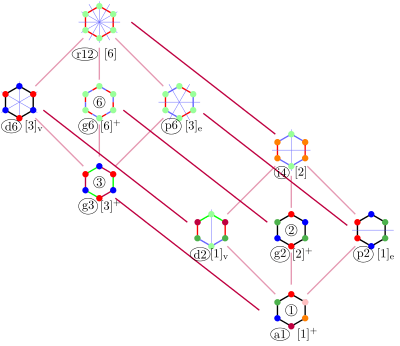Example subgroups from a hexagonal dihedral symmetry

D1 is isomorphic to Z2, the cyclic group of order 2.

D2 is isomorphic to K4, the Klein four-group.

D1 and D2 are exceptional in that:

• D1 and D2 are the only abelian dihedral groups. Otherwise, Dn is non-abelian.
• Dn is a subgroup of the symmetric group Sn for n ≥ 3. Since 2n > n! for n = 1 or n = 2, for these values, Dn is too large to be a subgroup.
• The inner automorphism group of D2 is trivial, whereas for other even values of n, this is Dn / Z2.

The cycle graphs of dihedral groups consist of an n-element cycle and n 2-element cycles. The dark vertex in the cycle graphs below of various dihedral groups represents the identity element, and the other vertices are the other elements of the group. A cycle consists of successive powers of either of the elements connected to the identity element.

Cycle graphs
D1 = Z2 D2 = Z22 = K4 D3 D4 D5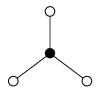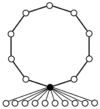D6 = D3 × Z2 D7 D8 D9 D10 = D5 × Z2
D3 = S3 D4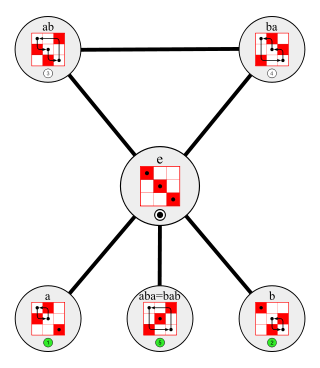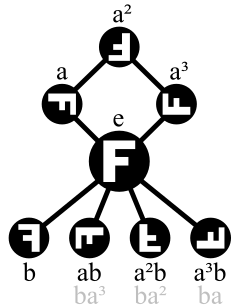## The dihedral group as symmetry group in 2D and rotation group in 3D

An example of abstract group Dn, and a common way to visualize it, is the group of Euclidean plane isometries which keep the origin fixed. These groups form one of the two series of discrete point groups in two dimensions. Dn consists of n rotations of multiples of 360°/n about the origin, and reflections across n lines through the origin, making angles of multiples of 180°/n with each other. This is the symmetry group of a regular polygon with n sides (for n ≥ 3; this extends to the cases n = 1 and n = 2 where we have a plane with respectively a point offset from the "center" of the "1-gon" and a "2-gon" or line segment).

Dn is generated by a rotation r of order n and a reflection s of order 2 such thatIn geometric terms: in the mirror a rotation looks like an inverse rotation.

In terms of complex numbers: multiplication byand complex conjugation.

In matrix form, by settingand definingandforwe can write the product rules for  Dn as(Compare coordinate rotations and reflections.)

The dihedral group D2 is generated by the rotation r of 180 degrees, and the reflection s across the x-axis. The elements of D2 can then be represented as {e, r, s, rs}, where e is the identity or null transformation and rs is the reflection across the y-axis.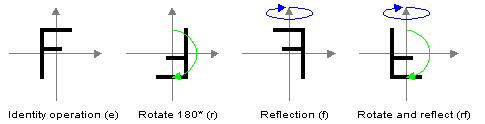The four elements of D2 (x-axis is vertical here)

D2 is isomorphic to the Klein four-group.

For n>2 the operations of rotation and reflection in general do not commute and Dn is not abelian; for example, in D4, a rotation of 90 degrees followed by a reflection yields a different result from a reflection followed by a rotation of 90 degrees.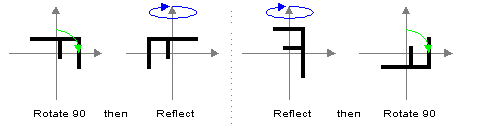D4 is nonabelian (x-axis is vertical here).

Thus, beyond their obvious application to problems of symmetry in the plane, these groups are among the simplest examples of non-abelian groups, and as such arise frequently as easy counterexamples to theorems which are restricted to abelian groups.

The 2n elements of Dn can be written as e, r, r2, … , rn−1, s, r s, r2s, … , rn−1s. The first n listed elements are rotations and the remaining n elements are axis-reflections (all of which have order 2). The product of two rotations or two reflections is a rotation; the product of a rotation and a reflection is a reflection.

So far, we have considered Dn to be a subgroup of O(2), i.e. the group of rotations (about the origin) and reflections (across axes through the origin) of the plane. However, notation Dn is also used for a subgroup of SO(3) which is also of abstract group type Dn: the proper symmetry group of a regular polygon embedded in three-dimensional space (if n ≥ 3). Such a figure may be considered as a degenerate regular solid with its face counted twice. Therefore, it is also called a dihedron (Greek: solid with two faces), which explains the name dihedral group (in analogy to tetrahedral, octahedral and icosahedral group, referring to the proper symmetry groups of a regular tetrahedron, octahedron, and icosahedron respectively).

## Properties

The properties of the dihedral groups Dn with n ≥ 3 depend on whether n is even or odd. For example, the center of Dn consists only of the identity if n is odd, but if n is even the center has two elements, namely the identity and the element rn/2 (with Dn as a subgroup of O(2), this is inversion; since it is scalar multiplication by −1, it is clear that it commutes with any linear transformation).

In the case of 2D isometries, this corresponds to adding inversion, giving rotations and mirrors in between the existing ones.

For n twice an odd number, the abstract group Dn is isomorphic with the direct product of Dn / 2 and Z2. Generally, if m divides n, then Dn has n/m subgroups of type Dm, and one subgroup ℤm. Therefore, the total number of subgroups of Dn (n  1), is equal to d(n) + σ(n), where d(n) is the number of positive divisors of n and σ(n) is the sum of the positive divisors of n. See list of small groups for the cases n  8.

The dihedral group of order 8 (D4) is the smallest example of a group that is not a T-group. Any of its two Klein four-group subgroups (which are normal in D4) has as normal subgroup order-2 subgroups generated by a reflection (flip) in D4, but these subgroups are not normal in D4.

### Conjugacy classes of reflections

All the reflections are conjugate to each other in case n is odd, but they fall into two conjugacy classes if n is even. If we think of the isometries of a regular n-gon: for odd n there are rotations in the group between every pair of mirrors, while for even n only half of the mirrors can be reached from one by these rotations. Geometrically, in an odd polygon every axis of symmetry passes through a vertex and a side, while in an even polygon there are two sets of axes, each corresponding to a conjugacy class: those that pass through two vertices and those that pass through two sides.

Algebraically, this is an instance of the conjugate Sylow theorem (for n odd): for n odd, each reflection, together with the identity, form a subgroup of order 2, which is a Sylow 2-subgroup (2 = 21 is the maximum power of 2 dividing 2n = 2[2k + 1]), while for n even, these order 2 subgroups are not Sylow subgroups because 4 (a higher power of 2) divides the order of the group.

For n even there is instead an outer automorphism interchanging the two types of reflections (properly, a class of outer automorphisms, which are all conjugate by an inner automorphism).

## Automorphism group

The automorphism group of Dn is isomorphic to the holomorph of ℤ/nℤ, i.e., to Hol(ℤ/nℤ) = {ax + b | (a, n) = 1} and has order nϕ(n), where ϕ is Euler's totient function, the number of k in 1, …, n − 1 coprime to n.

It can be understood in terms of the generators of a reflection and an elementary rotation (rotation by k(2π/n), for k coprime to n); which automorphisms are inner and outer depends on the parity of n.

• For n odd, the dihedral group is centerless, so any element defines a non-trivial inner automorphism; for n even, the rotation by 180° (reflection through the origin) is the non-trivial element of the center.
• Thus for n odd, the inner automorphism group has order 2n, and for n even (other than n = 2) the inner automorphism group has order n.
• For n odd, all reflections are conjugate; for n even, they fall into two classes (those through two vertices and those through two faces), related by an outer automorphism, which can be represented by rotation by π/n (half the minimal rotation).
• The rotations are a normal subgroup; conjugation by a reflection changes the sign (direction) of the rotation, but otherwise leaves them unchanged. Thus automorphisms that multiply angles by k (coprime to n) are outer unless k = ±1.

### Examples of automorphism groups

D9 has 18 inner automorphisms. As 2D isometry group D9, the group has mirrors at 20° intervals. The 18 inner automorphisms provide rotation of the mirrors by multiples of 20°, and reflections. As isometry group these are all automorphisms. As abstract group there are in addition to these, 36 outer automorphisms; e.g., multiplying angles of rotation by 2.

D10 has 10 inner automorphisms. As 2D isometry group D10, the group has mirrors at 18° intervals. The 10 inner automorphisms provide rotation of the mirrors by multiples of 36°, and reflections. As isometry group there are 10 more automorphisms; they are conjugates by isometries outside the group, rotating the mirrors 18° with respect to the inner automorphisms. As abstract group there are in addition to these 10 inner and 10 outer automorphisms, 20 more outer automorphisms; e.g., multiplying rotations by 3.

Compare the values 6 and 4 for Euler's totient function, the multiplicative group of integers modulo n for n = 9 and 10, respectively. This triples and doubles the number of automorphisms compared with the two automorphisms as isometries (keeping the order of the rotations the same or reversing the order).

The only values of n for which φ(n) = 2 are 3, 4, and 6, and consequently, there are only three dihedral groups that are isomorphic to their own automorphism groups, namely D3 (order 6), D4 (order 8), and D6 (order 12).

### Inner automorphism group

The inner automorphism group of Dn is isomorphic to:

• Dn if n is odd;
• Trivial if n = 2;
• Dn / Z2 if n is even and n > 2.

## Generalizations

There are several important generalizations of the dihedral groups:Wikimedia Commons has media related to Dihedral groups.